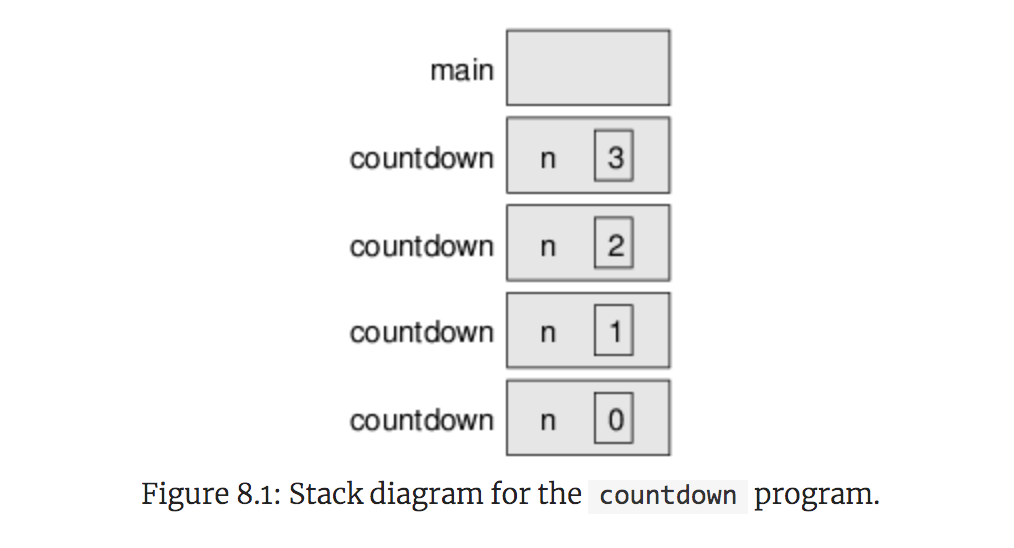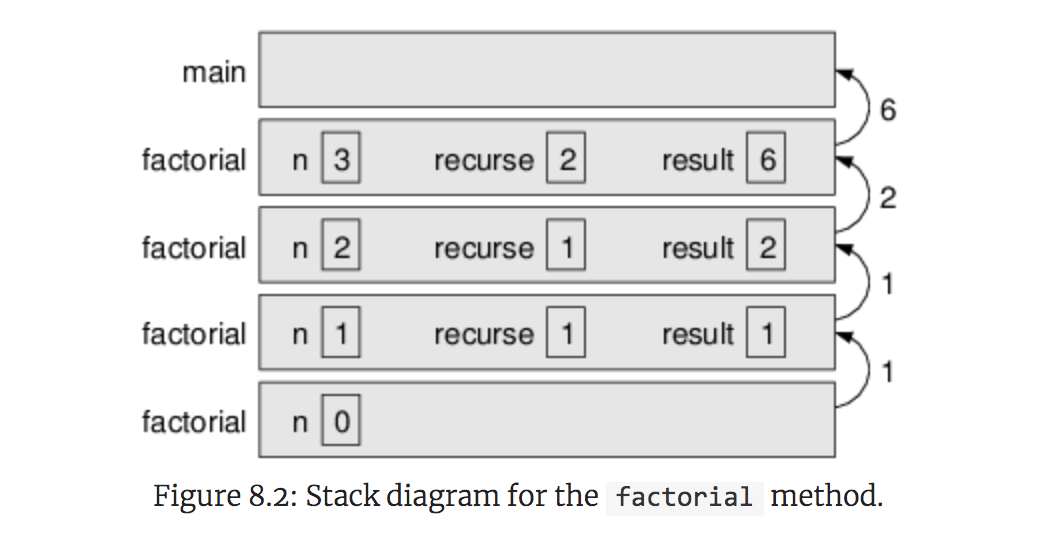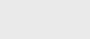3年前 (2020-10-04) |   抢沙发  1190

## 递归Void方法

``````public static void countdown(int n) {
if (n == 0) {
System.out.println("Blastoff!");
} else {
System.out.println(n);
countdown(n - 1);
}
}``````

``````倒计时的执行从n==3开始，由于n不是0，它显示值3，然后调用自己。。。

倒计时的执行从n==2开始，由于n不是0，它显示值2，然后调用自己。。。

倒计时的执行从n==1开始，由于n不是0，它显示值1，然后调用自己。。。

倒计时的执行从n==0开始，由于n是0，它显示单词Blastoff！然后回来。

得到n==1的倒计时返回。

得到n==2的倒计时返回。

``````3
2
1
Blastoff!``````

``````public static void newLine() {
System.out.println();
}

public static void threeLine() {
newLine();
newLine();
newLine();
}``````

``````public static void nLines(int n) {
if (n > 0) {
System.out.println();
nLines(n - 1);
}
}
``````

## 递归堆栈图``````public static void forever(String s) {
System.out.println(s);
forever(s);
}``````

### 值返回方法

``````0！=1

n！=n·（n−1）！``````

``````public static int factorial(int n) {
return 0;  // stub
}``````

``````public static int factorial(int n) {
if (n == 0) {
return 1;
}
return 0;  // stub
}``````

``````public static int factorial(int n) {
if (n == 0) {
return 1;
}
int recurse = factorial(n - 1);
int result = n * recurse;
return result;
}``````

``````由于3不是0，我们跳过第一个分支，计算n−1的阶乘。。。

因为2不是0，我们跳过第一个分支，计算n−1的阶乘。。。

由于1不是0，我们跳过第一个分支，计算n−1的阶乘。。。

因为0是0，所以我们获取第一个分支并立即返回值1。

返回值（1）乘以n，即1，然后返回结果。

返回值（1）乘以n，即2，然后返回结果。## 信仰的飞跃

``````public static boolean isSingleDigit(int x) {
return x > -10 && x < 10;
}``````

``````public static int factorial(int n) {
if (n == 0) {
return 1;
}
return n * factorial(n - 1);
}
``````

``````0！=1

n！=n·（n−1）！``````

``````fibonacci(1) = 1
fibonacci(2) = 1
fibonacci(n) = fibonacci(n−1) + fibonacci(n−2)``````

``````public static int fibonacci(int n) {
if (n == 1 || n == 2) {
return 1;
}
return fibonacci(n - 1) + fibonacci(n - 2);
}``````

## 递归计数

1. 检查基本情况
2. 显示某些内容
3. 进行递归调用

``````public static void countup(int n) {
if (n == 0) {
System.out.println("Blastoff!");
} else {
countup(n - 1);
System.out.println(n);
}
}``````

``````Blastoff!
1
2
3``````### 注册